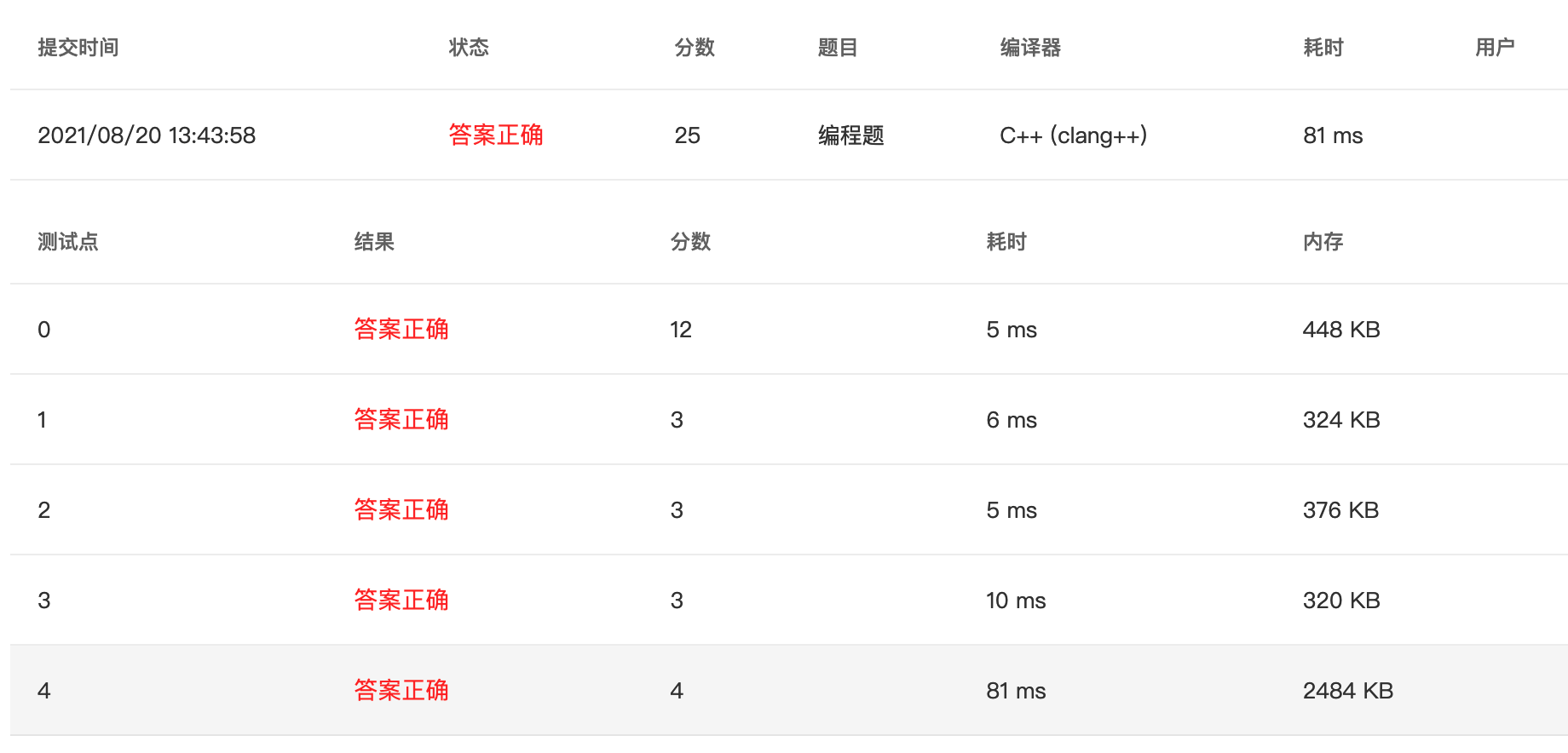# PTA甲级 1063 Set Similarity (C++)

2021/8/20 13:44:24 浏览：

Given two sets of integers, the similarity of the sets is defined to be N c / N t × 100 N_c/N_t ×100% , where N c N_c is the number of distinct common numbers shared by the two sets, and N t N_t is the total number of distinct numbers in the two sets. Your job is to calculate the similarity of any given pair of sets.

## Input Specification:

Each input file contains one test case. Each case first gives a positive integer N ( ≤ 50 ) N (≤50) which is the total number of sets. Then N N lines follow, each gives a set with a positive M ( ≤ 1 0 4 ) M (≤10^4) and followed by M M integers in the range [ 0 , 1 0 9 ] [0,10^9] . After the input of sets, a positive integer K ( ≤ 2000 ) K (≤2000) is given, followed by K K lines of queries. Each query gives a pair of set numbers (the sets are numbered from 1 to N N ). All the numbers in a line are separated by a space.

## Output Specification:

For each query, print in one line the similarity of the sets, in the percentage form accurate up to 1 decimal place.

## Sample Input:

3
3 99 87 101
4 87 101 5 87
7 99 101 18 5 135 18 99
2
1 2
1 3


## Sample Output:

50.0%
33.3%


## Solution:

// Talk is cheap, show me the code
// Created by Misdirection 2021-08-20 12:53:58

#include <iostream>
#include <vector>
#include <unordered_set>

using namespace std;

int main(){
int n;
scanf("%d", &n);
vector<unordered_set<int>> nums(n);

for(int i = 0; i < n; ++i){
int size;
scanf("%d", &size);

for(int j = 0; j < size; ++j){
int tmp;
scanf("%d", &tmp);

nums[i].insert(tmp);
}
}

int query;
scanf("%d", &query);

for(int i = 0; i < query; ++i){
int a, b;
scanf("%d %d", &a, &b);

int common = 0;
int total = nums[a - 1].size();

for(auto it = nums[b - 1].begin(); it != nums[b - 1].end(); ++it){
if(nums[a - 1].find(*it) != nums[a - 1].end()) common++;
else total++;
}

printf("%.1f%%\n", 100.0 * common / total);

}

return 0;
}